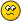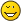# Tetration Forum

You're currently viewing a stripped down version of our content. View the full version with proper formatting.
Pages: 1 2
Hi.

Here I'll present some results of my playing around with the Borel summation method to extend tetration to fractional, real, and complex heights.

Borel sums

http://en.wikipedia.org/wiki/Borel_summation
http://mathworld.wolfram.com/Borel-RegularizedSum.html
http://arxiv.org/pdf/hep-th/9206074

(the third also discusses generalizations of the method)

Take

$$\sum_{n=0}^{\infty} a_n z^n$$

a formal power series. The Borel sum is given by

$$\sum_{n=0}^{\infty} a_n z^n \stackrel{pseudo}{=} \int_{0}^{\infty} e^{-zt} \left(\sum_{n=0}^{\infty} \frac{a_n}{n!} t^n\right) dt$$

(Caveat here: Wikipedia mentions $$a_{n+1}$$ instead of $$a_n$$, however MathWorld and the other paper use the latter. The latter will be used here as well.)

provided the sum inside can be continued analytically to $$[0, \infty)$$ and it decays fast enough there to yield convergence of the integral.

It is possible to set up a higher-order method with order $$r$$ via repeated application, yielding

$$\sum_{n=0}^{\infty} a_n \stackrel{pseudo}{=} \underbrace{\int_{0}^{\infty} \cdots \int_{0}^{\infty}}_{r} e^{-t_1 -t_2 \cdots -t_r} \left(\sum_{n=0}^{\infty} \frac{a_n}{(n!)^r} t_1^n t_2^n \cdots t_r^n \right) dt_1 \cdots dt_r$$

Application to tetration

We take Faulhaber's formula for the continuum sum:

$$\sum_{n=0}^{z-1} f(n) = \sum_{k=1}^{\infty} b_k z^k$$

where

$$b_k = \sum_{n=1}^{\infty} \frac{f^{(n-1)}(0)}{n!} {n \choose k} B_{n-k},\ B_1 = -\frac{1}{2}$$

and $$f(z)$$ is an analytic function, whose continuum sum we desire. The problem with this formula is it does not converge for any analytic function $$f(z)$$. It only converges if $$f(z)$$ is entire and is of exponential type of less than $$2\pi$$.

However, in some cases, it may still be possible to interpret the above formula as giving a continuum sum, through the employment of divergent summation techniques, such as the Cesaro summation, Euler summation, Abel summation, Borel summation, Ramanujan summation (not sure about that one since it would seem to imply using fractional derivatives which are nasty stuff), etc. Here though, we'll try it with Borel summation, giving

$$b_k \stackrel{pseudo}{=} \underbrace{\int_{0}^{\infty} \cdots \int_{0}^{\infty}}_{r} e^{-t_1 -t_2 \cdots -t_r} \left(\sum_{n=1}^{\infty} \frac{f^{(n-1)}(0)}{(n!)^{r+1}} {n \choose k} B_{n-k} t_1^n t_2^n \cdots t_r^n \right) dt_1 \cdots dt_r$$

Now we can use Ansus' continuum sum formula:

$$\log_b\left(\frac{\mathrm{tet}'_b(z)}{\mathrm{tet}'_b(0) \log(b)^z}\right) = \sum_{n=0}^{z-1} \mathrm{tet}_b(n)$$.

Numerical algorithm

We could evaluate the Borel summation numerically via numerical integration, however this is a very expensive task. Instead, we use the following approach:

$$\int_{0}^{\infty} e^{-t} \left(\sum_{n=0}^{\infty} \frac{a_n}{n!} t^n\right) dt \approx \int_{0}^{A} e^{-t} \left(\sum_{n=0}^{\infty} \frac{a_n}{n!} t^n\right) dt = \int_{0}^{A} \left(\sum_{n=0}^{\infty} \frac{a_n}{n!} e^{-t} t^n\right) dt = \sum_{n=0}^{\infty} \frac{a_n}{n!} \left(\int_{0}^{A} e^{-t} t^n dt\right) = \sum_{n=0}^{\infty} \frac{a_n}{n!} \gamma(A, n+1)$$.

Thus we get the formula

$$\sum_{n=0}^{\infty} a_n \stackrel{pseudo}{=} \lim_{A \rightarrow \infty} \sum_{n=0}^{\infty} a_n \frac{\gamma(A, n+1)}{\Gamma(n+1)}$$

which is much easier to compute with. For higher orders,

$$\sum_{n=0}^{\infty} a_n \stackrel{pseudo}{=} \lim_{A \rightarrow \infty} \sum_{n=0}^{\infty} a_n \left(\frac{\gamma(A, n+1)}{\Gamma(n+1)}\right)^r$$.

Thus for Faulhaber's formula, we have

$$b_k \stackrel{pseudo}{=} \lim_{A \rightarrow \infty} \sum_{n=1}^{\infty} \frac{f^{(n-1)}(0)}{n!} {n \choose k} B_{n-k} \left(\frac{\gamma(A, n+1)}{\Gamma(n+1)}\right)^r$$.

To create a numerical estimate for tetration, we apply the iterating Ansus formula

$$\mathrm{tet}_b(z) = \mathrm{tet}'_b(0) \int_{-1}^{z} \exp_b\left(\log(b)^w \sum_{n=0}^{w-1} \mathrm{tet}_b(n)\right) dw$$.

to a Taylor series at 0. The coefficient $$\mathrm{tet}'_b(0)$$ is acquired by normalizing after each iteration so that the approximation equals 1 at $$z = 0$$.

Investigation

To test the method, I implemented the above algorithm in PARI/GP (which has good power series manipulation and arbitrary precision systems), and ran it. The tests were done with base $$e$$.

Using 28 terms and 38 decimals of precision, first-order Borel summation and $$A = 20$$, I get a residual ($$\exp(F(-1/2)) - F(1/2)$$, where $$F$$ is the approximation) of magnitude $$6 \times 10^{-7}$$ and $$^{1/2} e \approx 1.646357$$. If I bump up the terms and decimals to 48 and $$A$$ to 24, it seems I need a higher order Borel summation, using order 2 gives a residual of $$1 \times 10^{-7}$$ and $$^{1/2} e \approx 1.6463562$$. Taking it to 128 terms, 128 decimals, and $$A = 28$$, I get a residual of order $$1 \times 10^{-8}$$ and $$^{1/2} e \approx 1.64635424$$. Precision/term requirements seem to get steep fast, not sure if I can push more digits out of this one, if I need higher order Borel summation, or even if it "really" does converge (in that case, there's those "generalized" Borel methods mentioned in that one paper but these are much, much slower numerically.).[/tex].

Experiment suggestion: The best value I got out of this, 1.64635424 for $$^{1/2} e$$ looks like that obtained from Kouznetsov's Cauchy-integral approach, and maybe also Robbins' tetration (Abel solution). I'd like to be able to have tons of Taylor terms precomputed from that approach (maybe 100 or more) to run though the Borel summation to see if the tetration really can be Borel-continuum-summed, however my current Cauchy integral code isn't fast enough to compute them all.

With the best approximation, I get

Program

The following PARI/GP code implements this numerical approximation technique. Feel free to play around with this.

Code:
base = exp(1); pdegree = 48; \ps 49; /* Bernoulli number B_n */ bernoulli(n) = if(n==0, 1, (-1)^(n+1) * n * zeta(1 - n)); /* Faulhaber kth term sum term */ faulhterm(n, k, polyn) = polcoeff(polyn, n-1)/n * binomial(n, k) * bernoulli(n-k); /* Do Borel summation of truncated series polyn. ubound1 and ubound 2 should be "close to real infinity". */ borelsum(terms, ubound, order) = {         local(maxterms = matsize(terms));         return(sum(k=0,maxterms-1,(terms[k+1]*incgamc(k+1, ubound)^order)/((k!)^order))); } /* Use Borel summation to build up continuum sum */ ContSumPoly(polyn, ubound, order) = {         local(outpolyn = 0);         local(nterms = poldegree(polyn)+1);         local(bernterms = vector(nterms));         for(k=0,poldegree(polyn),\             for(n=1,poldegree(polyn),bernterms[n]=faulhterm(n, k, polyn));\             outpolyn=outpolyn+(borelsum(bernterms, ubound, order)*x^k);            );         return(outpolyn - subst(outpolyn, x, 0)); } /* Compute exp of a power series */ exppoly(p) = truncate(base^p); /* Multiply by log(base)^x */ mullogb(p) = { local(multpoly = truncate(log(base)^x)*p, outp = 0);                for(i=0,pdegree,outp=outp+polcoeff(multpoly,i,x)*x^i);                return(outp); } /* Compute definite integral from -1 to x of a power series */ defint(p) = { local(integ=intformal(p)); return(integ - subst(integ, x, -1)); } /* Do one iteration of the formula */ iterate(p, ubound, order) = defint(mullogb(exppoly(ContSumPoly(p, ubound, order)))); /* Normalized iteration */ iternorm(p, ubound, order) = { local(pi=iterate(p, ubound, order)); return(pi/polcoeff(pi, 0)); } /* N iterations on an initial guess */ niters(n, p, ubound, order) = { local(result=p); for(i=1,n,result=iterate(result,ubound,order)); return(result); } /* N normalized iterations on an initial guess */ nnormiters(n, p, ubound, order) = { local(result=p); for(i=1,n,result=iternorm(result,ubound,order)); return(result); } /* Use polynomial */ sumpoly(p, evalpoint) = subst(p, x, evalpoint); /* Compute residual of tetration */ residual(p) = log(sumpoly(p, 0.5))/log(base) - sumpoly(p, -0.5); /* Real tetration */ tetcrit(X) = sumpoly(tetpoly, X) realtet(X) = {        if(X < -0.5, return(log(realtet(X+1))/log(base)));        if(X > 0.5, return(base^realtet(X-1)));        return(tetcrit(X)); }
ok.but I got "tetpoly" or a group of numbers and not 1 real or complex number correct only because "tetpoly" is empty.(03/24/2010, 01:11 AM)mike3 Wrote: [ -> ]$$\sum_{n=0}^{\infty} a_n \stackrel{pseudo}{=} \lim_{A \rightarrow \infty} \sum_{n=0}^{\infty} a_n \frac{\gamma(A, n+1)}{\Gamma(n+1)}$$

Hm, this works? This is really amazing, considering that
$$g_n=\frac{\gamma(A, n+1)}{\Gamma(n+1)}\to 1$$ for $$A\to\infty$$
In this case indeed summation and limes is not swapable.

Cool investigations!
(03/24/2010, 01:56 PM)nuninho1980 Wrote: [ -> ]ok.but I got "tetploy" or a group of numbers and not 1 real or complex number correct only because "tetpoly" is empty.To use it, try setting tetpoly with an initial guess first, e.g. tetpoly = 1 or tetpoly = 1 + 1.09*x. Then apply tetpoly = iternorm(tetpoly, A, order) (order is the order of the Borel summation.) some times to improve the accuracy. You can use nnormiters to do a batch of normalized iterations.
(03/24/2010, 05:57 PM)bo198214 Wrote: [ -> ]Hm, this works? This is really amazing, considering that
$$g_n=\frac{\gamma(A, n+1)}{\Gamma(n+1)}\to 1$$ for $$A\to\infty$$
In this case indeed summation and limes is not swapable.

Cool investigations!

I'm not entirely sure if it really works, though it seems to. And yeah, if the limit was inside the summation sign it wouldn't go. The integral can be written as the limit of a finite one. This limit sign (not written in the derivation) always stays outside the summation sign.
(03/24/2010, 07:39 PM)mike3 Wrote: [ -> ]To use it, try setting tetpoly with an initial guess first, e.g. tetpoly = 1 or tetpoly = 1 + 1.09*x. Then apply tetpoly = iternorm(tetpoly, A, order) (order is the order of the Borel summation.) some times to improve the accuracy. You can use nnormiters to do a batch of normalized iterations.
I got error "inegame: incorrect... in gtofp." and I don't know how do I do this code because iternorm(p, ubound, order) is different to iternorm(tetpoly, A, order).
(03/24/2010, 08:56 PM)nuninho1980 Wrote: [ -> ]
(03/24/2010, 07:39 PM)mike3 Wrote: [ -> ]To use it, try setting tetpoly with an initial guess first, e.g. tetpoly = 1 or tetpoly = 1 + 1.09*x. Then apply tetpoly = iternorm(tetpoly, A, order) (order is the order of the Borel summation.) some times to improve the accuracy. You can use nnormiters to do a batch of normalized iterations.
I got error "inegame: incorrect... in gtofp." and I don't know how do I do this code because iternorm(p, ubound, order) is different to iternorm(tetpoly, A, order).

p = polynomial to apply to
ubound = variable called "A" in discussion
order = variable called "r" in discussion

I'm not sure what you tried to put in there, it should have worked. Did you try something like

Code:
tetpoly = 1 tetpoly = iternorm(tetpoly, 20, 2) (repeat the last one as many times as you want)

(that would be for A = 20, r = 2)

(03/24/2010, 09:43 PM)mike3 Wrote: [ -> ]I got error "inegame: incorrect... in gtofp." and I don't know how do I do this code because iternorm(p, ubound, order) is different to iternorm(tetpoly, A, order).

p = polynomial to apply to
ubound = variable called "A" in discussion
order = variable called "r" in discussion

I'm not sure what you tried to put in there, it should have worked. Did you try something like

Code:
tetpoly = 1 tetpoly = iternorm(tetpoly, 20, 2) (repeat the last one as many times as you want)

(that would be for A = 20, r = 2)

[/quote]

yes. I tried to increase "A" and "r" and still same result. but e^^0.5 = 1.5 only!?? incorrect. how do you do for better result?
(03/24/2010, 10:33 PM)nuninho1980 Wrote: [ -> ]yes. I tried to increase "A" and "r" and still same result. but e^^0.5 = 1.5 only!?? incorrect. how do you do for better result?

I find this really bizarre... What version of Pari/GP are you using? I use 2.3.4.

Oh wait, did you just do one iteration and one iteration only? That might be the problem. Try running the command several times. You can also use the nnormiters routine. I.e. tetpoly = nnormiters(5, tetpoly, 20, 2) is the same as running tetpoly = iternorm(tetpoly, 20, 2) 5 times.
(03/25/2010, 02:19 AM)mike3 Wrote: [ -> ]
(03/24/2010, 10:33 PM)nuninho1980 Wrote: [ -> ]yes. I tried to increase "A" and "r" and still same result. but e^^0.5 = 1.5 only!?? incorrect. how do you do for better result?

I find this really bizarre... What version of Pari/GP are you using? I use 2.3.4.

Oh wait, did you just do one iteration and one iteration only? That might be the problem. Try running the command several times. You can also use the nnormiters routine. I.e. tetpoly = nnormiters(5, tetpoly, 20, 2) is the same as running tetpoly = iternorm(tetpoly, 20, 2) 5 times.
I have same lastest version running win xp-sp3.I will try...
Pages: 1 2